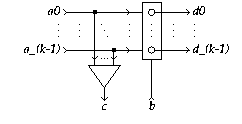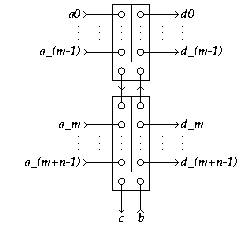EDIS: Guide | FAQ | New | Search | Bibliography | Index | Feedback

# k-Call

## Specifications

### Informal

A k-Call (k>0) has k+1 input terminals a_i (0<=i<k) and b, and k+1 output terminals c and d_i (0<=i<k). A signal appearing on either of the a_i terminals will cause a signal to be produced at terminal c. The combination of a signal at terminal a_i and terminal b will cause a signal to be produced at terminal d_i. The environment must guarantee mutual exclusion of the a_i-inputs.

### XDI

Schematic diagram for a k-Call:[Zoom|FIG]

We provide XDI specifications for the 3-call:

Specification in XDI model.

the 4-call:

Specification in XDI model.

and the 5-call:

Specification in XDI model.

(Not available for general k.)

### Verdect

Parameterized definitions are not possible in VERDECT, but this sketch gives the general idea:

Specification in Verdect:

```
define CALL[k]( a0?, ..., a(k-1)?, b?, c!, d0!, ..., d(k-1)! ) =
pref *[ (a0? | ... | a(k-1)?); c! ]
|| pref *[ (a0? || b?); d0!
| ...
| (a(k-1)? || b?); d(k-1)!
]
end
```

### DI Algebra

Pure DI Algebra does not allow a generalized specification. The following specification therefore needs instantiation before interpretation:

Specification in DI Algebra:

```CALL(k) = [(,i:0<= i < k: a_k? -> c!; C(i,k))]
C(i,k)  = [ b? -> d_i!; CALL(k), (,j: 0<= j < k: a_j? -> a_j? -> CHAOS)]

```

We provide DI Algebra specifications for

## Properties

As an example we give the XDI Report for the case k=3.

The roles of subscripts i can be permuted (on a_i and d_i simultaneously).

The k-Call satisfies Rules Y' and Z^out, but not Z^inp (choice between a_i inputs).

## Implementations

### DI Decompositions

1. A 2-Call is a Call.
2. A k-Call can be implemented with k Fork, a k-Merge and a k-Latch (equ):[Zoom|FIG]
3. An (m+n)-Call can be implemented with an m-Call and an (n+1)-Call (equ):[Zoom|FIG]

As an example we composed two 5-calls to form a 9-call. The Ludwig verification script of this implementation..

### Using Boolean Gates

No information available

### Using Transistors

No information available

## Generalizations

The k-Call can be generalized to an mxn-Decision Call, which merges m mutually exclusive inputs and also combines them with n mutually exclusive inputs, reporting the combination as one among mn outputs. A k-Call is an mxn-Decision-Wait with n=1.

## Miscellaneous

No information available